# Source Separation with Sparsity¶

Important: Please read the installation page for details about how to install the toolboxes. $\newcommand{\dotp}{\langle #1, #2 \rangle}$ $\newcommand{\enscond}{\lbrace #1, #2 \rbrace}$ $\newcommand{\pd}{ \frac{ \partial #1}{\partial #2} }$ $\newcommand{\umin}{\underset{#1}{\min}\;}$ $\newcommand{\umax}{\underset{#1}{\max}\;}$ $\newcommand{\umin}{\underset{#1}{\min}\;}$ $\newcommand{\uargmin}{\underset{#1}{argmin}\;}$ $\newcommand{\norm}{\|#1\|}$ $\newcommand{\abs}{\left|#1\right|}$ $\newcommand{\choice}{ \left\{ \begin{array}{l} #1 \end{array} \right. }$ $\newcommand{\pa}{\left(#1\right)}$ $\newcommand{\diag}{{diag}\left( #1 \right)}$ $\newcommand{\qandq}{\quad\text{and}\quad}$ $\newcommand{\qwhereq}{\quad\text{where}\quad}$ $\newcommand{\qifq}{ \quad \text{if} \quad }$ $\newcommand{\qarrq}{ \quad \Longrightarrow \quad }$ $\newcommand{\ZZ}{\mathbb{Z}}$ $\newcommand{\CC}{\mathbb{C}}$ $\newcommand{\RR}{\mathbb{R}}$ $\newcommand{\EE}{\mathbb{E}}$ $\newcommand{\Zz}{\mathcal{Z}}$ $\newcommand{\Ww}{\mathcal{W}}$ $\newcommand{\Vv}{\mathcal{V}}$ $\newcommand{\Nn}{\mathcal{N}}$ $\newcommand{\NN}{\mathcal{N}}$ $\newcommand{\Hh}{\mathcal{H}}$ $\newcommand{\Bb}{\mathcal{B}}$ $\newcommand{\Ee}{\mathcal{E}}$ $\newcommand{\Cc}{\mathcal{C}}$ $\newcommand{\Gg}{\mathcal{G}}$ $\newcommand{\Ss}{\mathcal{S}}$ $\newcommand{\Pp}{\mathcal{P}}$ $\newcommand{\Ff}{\mathcal{F}}$ $\newcommand{\Xx}{\mathcal{X}}$ $\newcommand{\Mm}{\mathcal{M}}$ $\newcommand{\Ii}{\mathcal{I}}$ $\newcommand{\Dd}{\mathcal{D}}$ $\newcommand{\Ll}{\mathcal{L}}$ $\newcommand{\Tt}{\mathcal{T}}$ $\newcommand{\si}{\sigma}$ $\newcommand{\al}{\alpha}$ $\newcommand{\la}{\lambda}$ $\newcommand{\ga}{\gamma}$ $\newcommand{\Ga}{\Gamma}$ $\newcommand{\La}{\Lambda}$ $\newcommand{\si}{\sigma}$ $\newcommand{\Si}{\Sigma}$ $\newcommand{\be}{\beta}$ $\newcommand{\de}{\delta}$ $\newcommand{\De}{\Delta}$ $\newcommand{\phi}{\varphi}$ $\newcommand{\th}{\theta}$ $\newcommand{\om}{\omega}$ $\newcommand{\Om}{\Omega}$

This numerical tour explore local Fourier analysis of sounds, and its application to source separation from stereo measurements.

In :
addpath('toolbox_signal')


## Sound Mixing¶

We load 3 sounds and simulate a stero recording by performing a linear blending of the sounds.

For Scilab users, it is safer to extend the stack size. For Matlab users this does nothing.

In :
extend_stack_size(4);


In :
n = 1024*16;
s = 3; % number of sound
p = 2; % number of micros
options.subsampling = 1;
x = zeros(n,3);


normalize the energy of the signals

In :
x = x./repmat(std(x,1), [n 1]);


We mix the sound using a |2x3| transformation matrix. Here the direction are well-spaced, but you can try with more complicated mixing matrices.

compute the mixing matrix

In :
theta = linspace(0,pi(),s+1); theta(s+1) = [];
theta(1) = .2;
M = [cos(theta); sin(theta)];


compute the mixed sources

In :
y = x*M';


Display of the sounds and their mix.

In :
clf;
for i=1:s
subplot(s,1,i);
plot(x(:,i)); axis('tight');
set_graphic_sizes([], 20);
title(strcat('Source #',num2str(i)));
end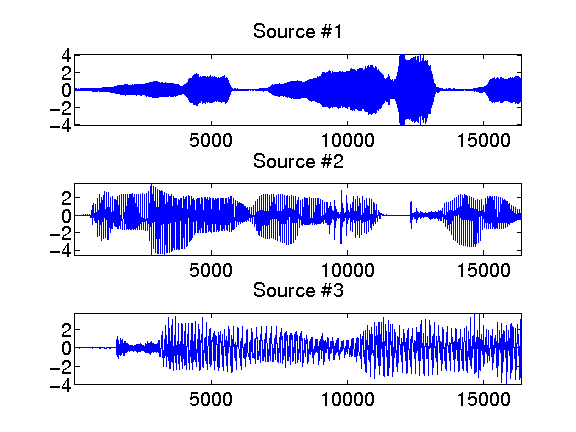Display of the micro output.

In :
clf;
for i=1:p
subplot(p,1,i);
plot(y(:,i)); axis('tight');
set_graphic_sizes([], 20);
title(strcat('Micro #',num2str(i)));
end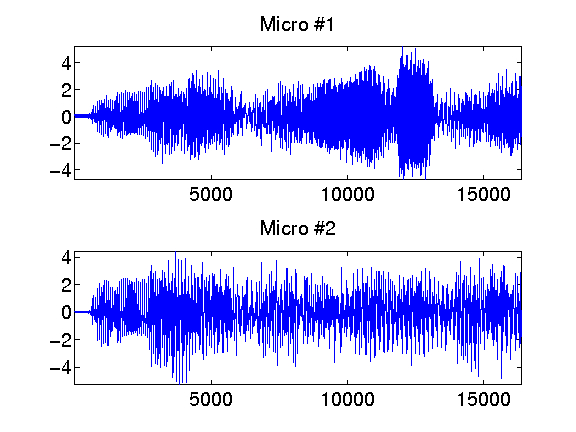## Local Fourier analysis of sound.¶

In order to perform the separation, one performs a local Fourier analysis of the sound. The hope is that the sources will be well-separated over the Fourier domain because the sources are sparse after a STFT.

First set up parameters for the STFT.

In :
options.n = n;
w = 128;   % size of the window
q = w/4;    % overlap of the window


Compute the STFT of the sources.

In :
clf; X = []; Y = [];
for i=1:s
X(:,:,i) = perform_stft(x(:,i),w,q, options);
subplot(s,1,i);
plot_spectrogram(X(:,:,i));
set_graphic_sizes([], 20);
title(strcat('Source #',num2str(i)));
end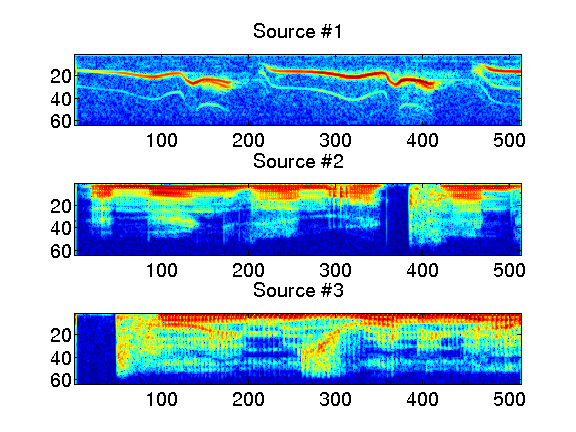Exercise 1

Compute the STFT of the micros, and store them into a matrix |Y|.

In :
exo1()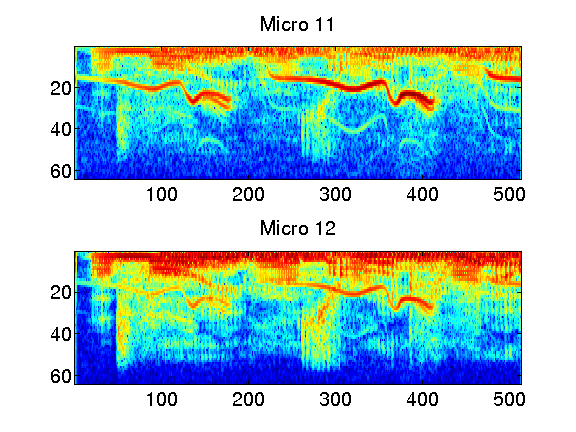In :
%% Insert your code here.


## Estimation of Mixing Direction by Clustering¶

Since the sources are quite sparse over the Fourier plane, the directions are well estimated by looking as the direction emerging from a point clouds of the transformed coefficients.

First we compute the position of the point cloud.

In :
mf = size(Y,1);
mt = size(Y,2);
P = reshape(Y, [mt*mf p]);
P = [real(P);imag(P)];


Then we keep only the 5% of points with largest energy.

Display some points in the original (spacial) domain.

number of displayed points

In :
npts = 6000;


display original points

In :
sel = randperm(n); sel = sel(1:npts);
clf;
plot(y(sel,1), y(sel,2), '.');
axis([-1 1 -1 1]*5);
set_graphic_sizes([], 20);
title('Time domain');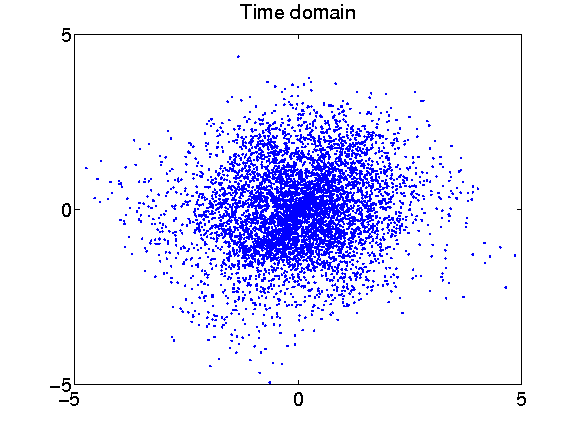Exercise 2

Display some points of |P| in the transformed (time/frequency) domain.

In :
exo2()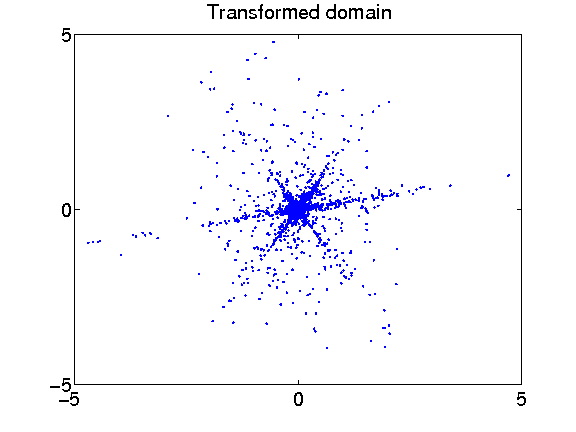In :
%% Insert your code here.


We compute the angle associated to each point over the transformed domain. The histograms shows the main direction of mixing.

In :
Theta = mod(atan2(P(:,2),P(:,1)), pi());


display histograms

In :
nbins = 100;
[h,t] = hist(Theta, nbins);
h = h/sum(h);
clf;
bar(t,h); axis('tight');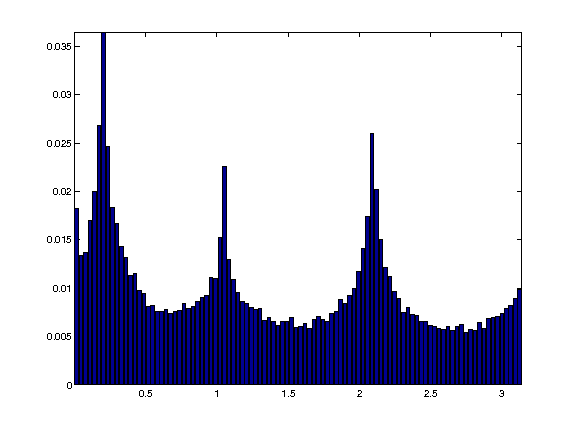Exercise 3

The histogram computed from the whole set of points are not peacked enough. To stabilize the detection of mixing direction, compute an histogram from a reduced set of point that have the largest amplitude. ompute the energy of each point xtract only a small sub-set ompute histogram isplay histograms

In :
exo3()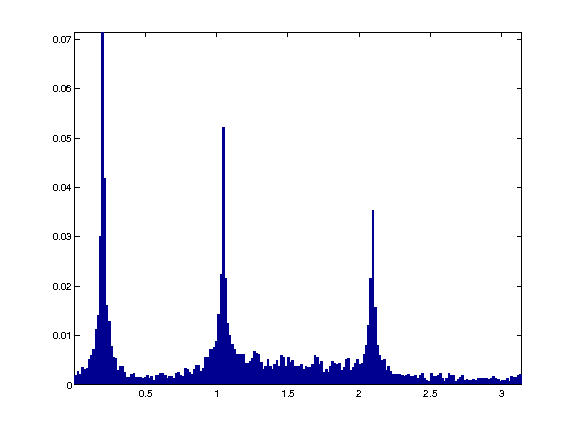In :
%% Insert your code here.


Exercise 4

Detect the direction |M1| approximating the true direction |M| by looking at the local maxima of the histogram. First detect the set of local maxima, and then keep only the three largest. ort in descending order

In :
exo4()

M =

0.9801    0.5000   -0.5000
0.1987    0.8660    0.8660

M1 =

0.9803    0.5010   -0.5028
0.1973    0.8655    0.8644


In :
%% Insert your code here.


## Separation of the Sources using Clustering¶

Once the mixing direction are known, one can project the sources on the direction.

We compute the projection of the coefficients Y on each estimated direction.

In :
A = reshape(Y, [mt*mf p]);


compute the projection of the coefficients on the directions

In :
C = abs( M1'*A' );


At each point |x|, the index |I(x)| is the direction which creates the largest projection.

I is the index of the closest source

In :
[tmp,I] = compute_max(C, 1);
I = reshape(I, [mf mt]);


An additional denoising is achieved by removing small coefficients.

do not take into account too small coefficients

In :
T = .05;
D = sqrt(sum( abs(Y).^2, 3));
I = I .* (D>T);


We can display the segmentation of the time frequency plane.

In :
clf;
imageplot(I(1:mf/2,:));
axis('normal');
set_colormap('jet');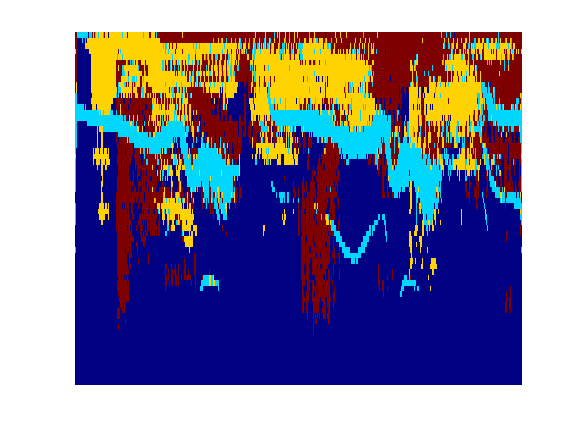The recovered coefficients are obtained by projection.

In :
Proj = M1'*A';
Xr = [];
for i=1:s
Xr(:,:,i) = reshape(Proj(i,:), [mf mt]) .* (I==i);
end


The estimated signals are obtained by inverting the STFT.

In :
for i=1:s
xr(:,i) = perform_stft(Xr(:,:,i),w,q, options);
end


One can display the recovered signals.

In :
clf;
for i=1:s
subplot(s,1,i);
plot(xr(:,i)); axis('tight');
set_graphic_sizes([], 20);
title(strcat('Estimated source #',num2str(i)));
end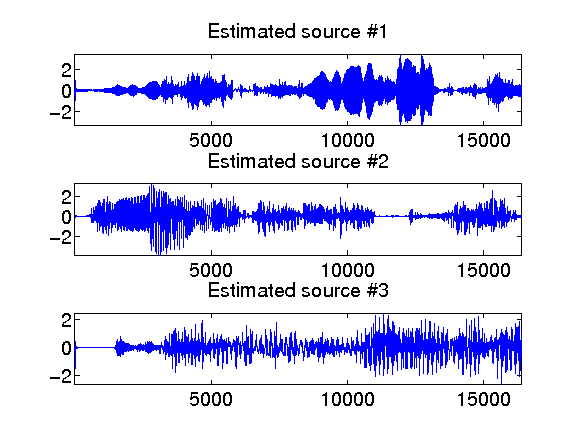One can listen to the recovered sources.

In :
i = 1;
sound(x(:,i),fs);
sound(xr(:,i),fs);